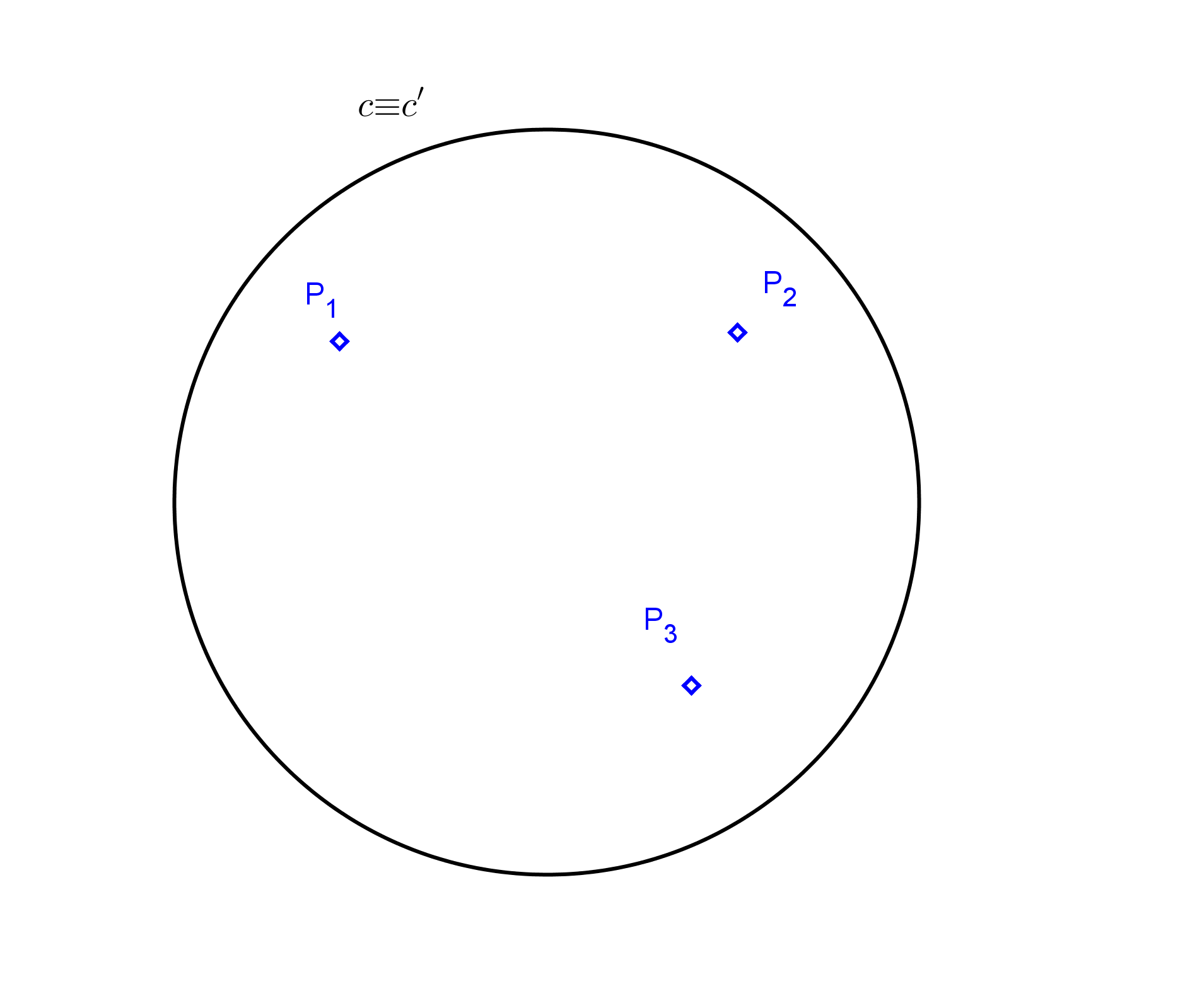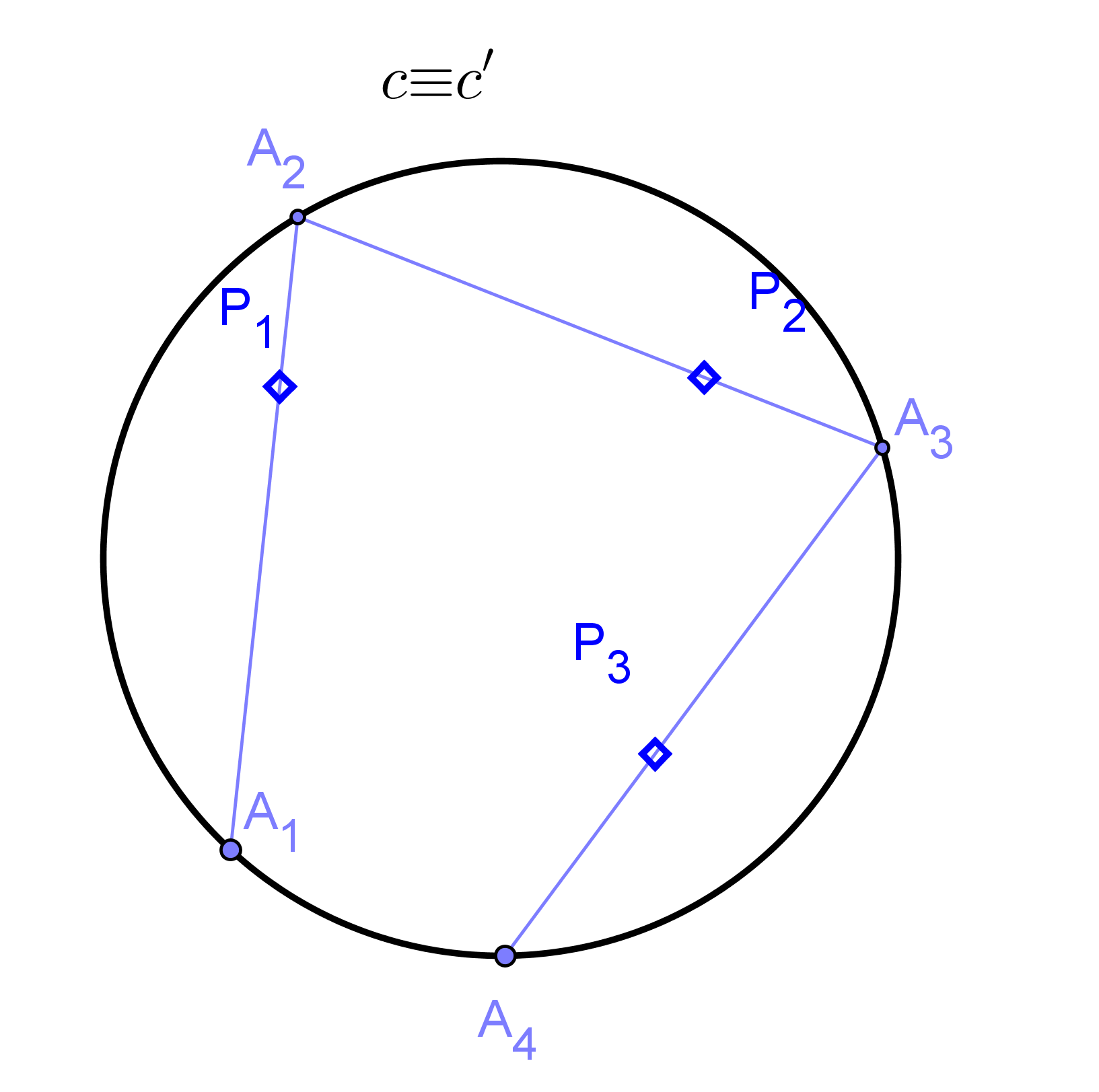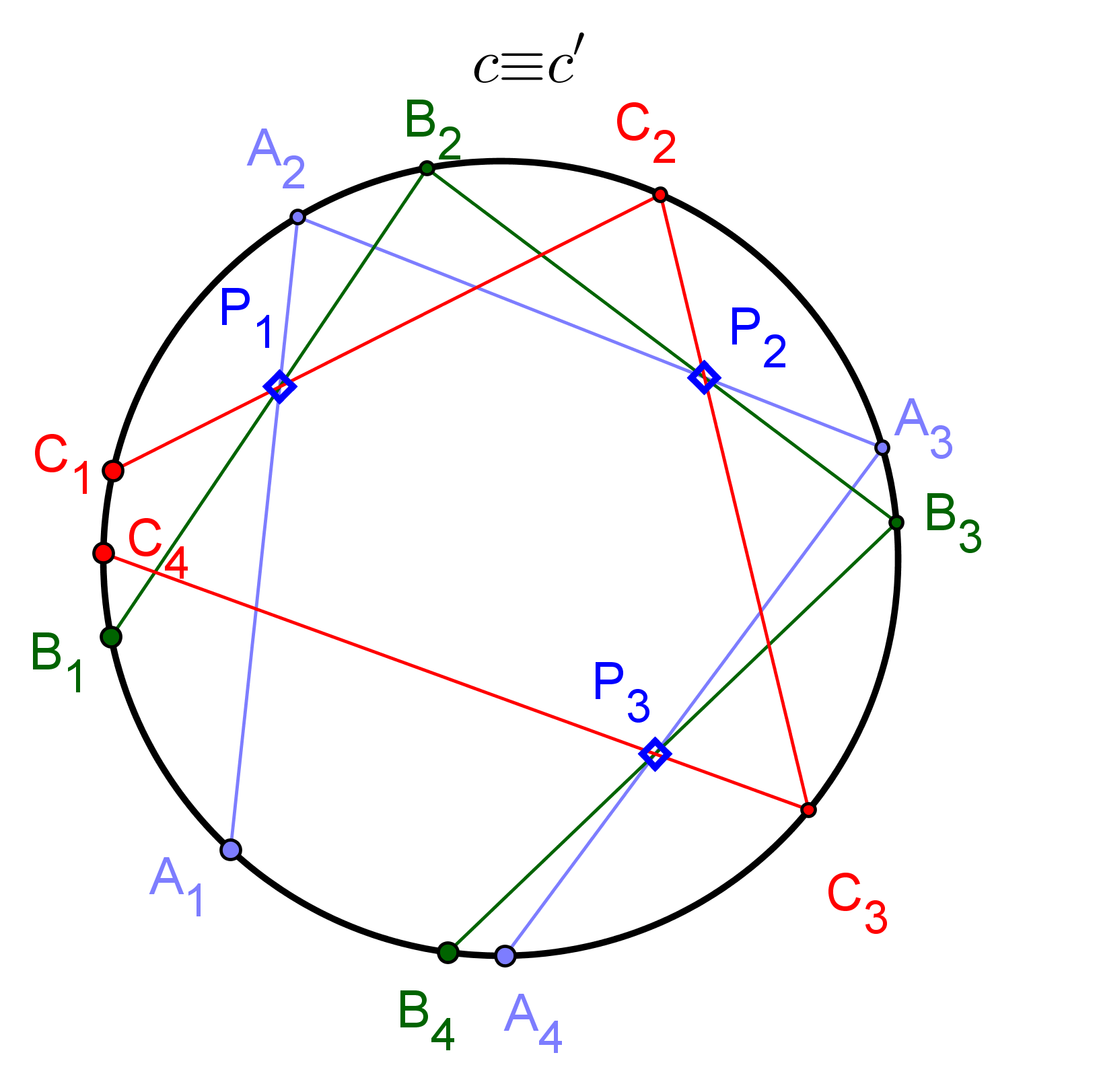# The false position method. Application of overlapping series of second order.The theoretical models of projective geometry can be proposing problems that are not of direct application. We will have that “dress up” therefore exercises to infer in the student further analysis and a transverse treatment of knowledge: Can I apply what they learn to solve this problem?.

This generalization of the application of concepts to solving various cases constitutes the last training stage in the learning of any discipline.

Professor Juan Alonso Alriols It presents us with an article with a proposal of exercise in projective geometry showing its strength, decorating it with a dynamic construction with GeoGebra, as used in another of his articles “Dynamic construction of a Tetrad of points“. A magnificent contribution that we will add to the set of themes of “Projective Geometry

## The false position method. Application of overlapping series of second order.

By Juan Alonso Alriols

After analyzing in detail the operations with overlapping series of second order, Let's see an example of application which does not consist in obtaining new tangent or points of contact a conical.The proposed problem is to find the triangle inscribed in a circumference whose sides pass through three given points (P1, P2, P3) as shown in the figure.To solve it we are going to take A point on the circumference and draw 3 chained segments respectively passing through P1, P2 and P3. As we have not successful to replace to1 in the correct position, We have obtained an "open triangle" in which to4 does not match A1.If I got to define two superimposed series of second order on the circumference c, the is double points, would be sought after points that would pass the solution triangle. As already established in the entrance overlapping series of second order, The projectivity between two overlapping series second order will be determined when we know three pairs of homologous points located on the same conical (A-A’, B-B’, C-C’). So we draw other two concatenations of segments from two points B1 and (C)1.Once we define the is c (A1, B1, C1) y c’ (A4, B4, C4), all that remains is to calculate the double points D1 and (D)2 to be found at the intersection of the projective axis with the Conic support based on second order as We studied beforehand.

Below you can see a dynamic construction of the problem made with Geogebra. At the bottom there are a few sliders that allow to move through the steps of the building leading to the solution. Also, You can move the points P1, A1, B1 and (C)1.

Finally we bring you a couple of questions. Is there solution of the problem for any position of the data? What are the minimum and maximum number of solutions? What is the position of the projective shaft with that number relationship? The previous construction would be valid if instead of a circle?, We have an ellipse?